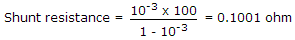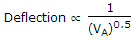# Electronics and Communication Engineering - Measurements and Instrumentation

### Exercise :: Measurements and Instrumentation - Section 4

1.

A 1 mA meter is to be converted to 1 A ammeter. The meter resistance is 100 Ω. The value of shunt resistance is

 A. 0.001 Ω B. 0.1001 Ω C. 100 Ω D. 1000 Ω

Explanation:.

2.

The use of thermocouple meters for ac measurement leads to a scale which is

 A. linear B. square law C. logarithmic D. exponential

Explanation:

Thermocouple meter is based on heating effect which varies as I2.

3.

Assertion (A): A low power factor wattmeter has special constructional features to ensure accurate measurement.

Reason (R): Extension of wattmeter range in dc circuit can be easily done by using current and potential transformer.

 A. Both A and R are true and R is correct explanation of A B. Both A and R are true but R is not correct explanation of A C. A is true R is false D. A is false R is true

Explanation:

CT and PT cannot be used in dc.

4.

The scale of dynamometer wattmeter is non linear.

 A. True B. False

Explanation:

It is linear.

5.

Magnetic deflection is inversely proportional to

 A. voltage B. (voltage)0.5 C. (voltage)1.5 D. (voltage)2

Explanation:where VA is the potential difference between anode and cathode.